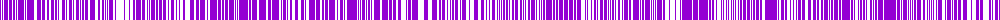# Gilles & Michiel De Mey

This is a common scenario — you have a lot of tasks that need to happen on a repeating interval. You might think `cron` is the obvious answer, but what if there are simply too many?

Using `cron` would run all of the tasks with the same interval at the same time, this is called the “top of the minute problem”.

To allow many tasks to be scheduled and spread within a fixed time window, and also guaranteeing that each task will always have the same offset, we can use hash functions.

We can use any hash that returns a 32-bit integer, I recommend `farmhash`, `murmurhash` or `xxhash` since these are fast, non-cryptographic hash functions with a low chance of collision.

In this example I’m using FarmHash, there’s a great Node package with native bindings that has great performance.

We’ll only need a few lines of code — let’s dig in.

``````// require the farmhash package
const farmhash = require('farmhash')

// Let's convert our time window to milliseconds,
// the smallest unit of time we can use
const TIME_WINDOW = 15 * 60 * 1000

// Calculate a random 32bit hash from an ID that uniquely
// identifies the task, this hash is guaranteed to be identical
// every time
const hash = farmhash.hash32(uid)

// Use the modulus operator to make sure our hash
// gets an integer assigned in the specified time window,
// this is the offset within the time window.
const offset = hash % TIME_WINDOW

// All that's left to do is to calculate the next occurrence
// of our schedule and add the offset.
const executeAt = getNextOccurrence() + offset
``````

We can use gnuplot to visualize the distribution of our offsets for a 1.000 random keys.

``````set terminal png size 1000,20
set yrange [0:1]
set format x ""
set format y ""
unset grid
unset key
unset tics
unset border
set lmargin 0
set rmargin 0
plot 'spread.csv' with impulses lw 0 notitle
``````

Which yields the following plot:As you can see the random distribution isn’t perfect, but good enough.